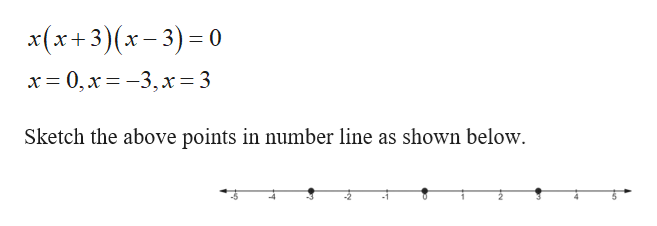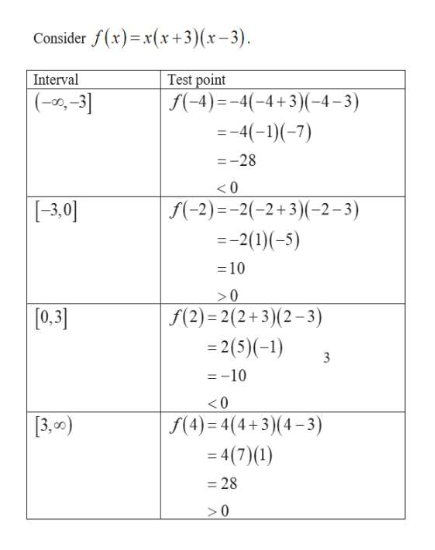# What would the solution set for x(x+3)(x−3)≤0 in interval notation?

Question
2 views

What would the solution set for x(x+3)(x−3)≤0 in interval notation?

check_circle

Step 1

Find the values of x where x=0.help_outlineImage Transcriptionclosex(x+3)(x-3)0 x 0,x-3,x= 3 Sketch the above points in number line as shown below fullscreen
Step 2

Find the interval the in...help_outlineImage TranscriptioncloseConsider f(x)x(x+3) (x-3) Test point -4)4(-4+3)-4-3) Interval (--31 -4(-1)(-7) =-28 < 0 [3,0] f(-2)=-2(-2+3)(-2-3) -2(1)(-5) 10 >0 f(2) 2(2+3)(2-3) =2(5)(-1) [0,3] 3 =-10 <0 3,0) f(4)=4(4+3)(4-3) 4(7)(1) 28 0 fullscreen

### Want to see the full answer?

See Solution

#### Want to see this answer and more?

Solutions are written by subject experts who are available 24/7. Questions are typically answered within 1 hour.*

See Solution
*Response times may vary by subject and question.
Tagged in

### Other